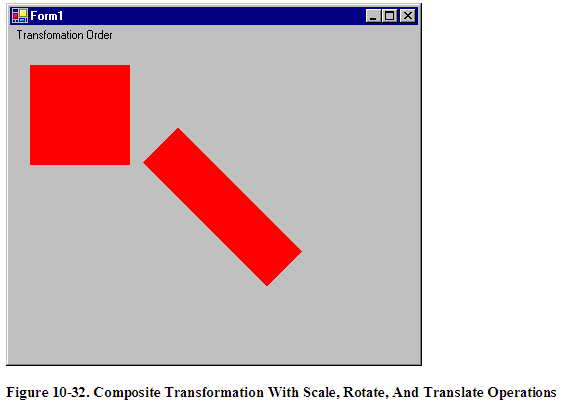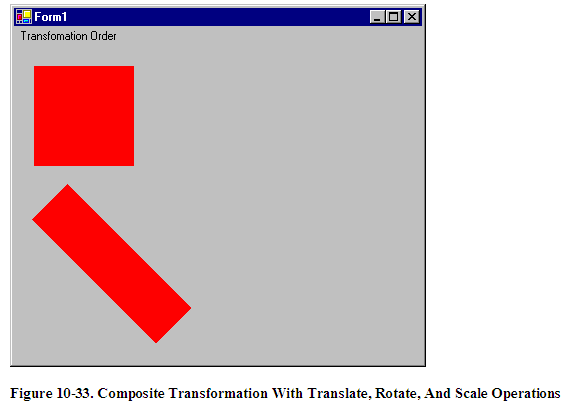# Significance of Transformation Order

The Matrix object can store a single transformation or a sequence of transformations. A sequence of transformations is called a composite transformation, which is a result multiplying the matrices of the individual transformations.

In a composite transformation, the order of the individual transformations is very important. Matrix operations are not cumulative. For example, Graphics -> Rotate -> Translate -> Scale -> Graphics operation will generate a different result than the Graphics -> Scale -> Rotate -> Translate -> Graphics operation. The main reason order is significant is that transformations like rotation and scaling are done with respect to the origin of the coordinate system. Scaling an object that is centered at the origin produces a different result than scaling an object that has been moved away from the origin. Similarly, rotating an object that is centered at the origin produces a different result than rotating an object that has been moved away from the origin.

The MatrixOrder enumeration, which is an argument to the transformation methods, represents the transformation order. It has two values: Append and Prepend. The Append value indicates that the new operation will be applied after the preceding operation, while the Prepend value indicates that the new operation will be applied before the preceding operation.

Now let's write an application to see the transformation order. We create a Windows application and add a MainMenu control to the form. We add three menu items to the MainMenu. We also add areference to the System.Drawing.Drawing2D namespace because we will use the MatrixOrder enumeration, which is defined there.
Listing 10-20 draws a rectangle before and after applying a scale, rotate, and translate sequence of transformations.

private void First_Click(object sender,System.EventArgs e)
{
// Create a Graphics object
Graphics g = this
.CreateGraphics();
g.Clear(
this
.BackColor);
// Create a Rectangle
Rectangle rect =
new
Rectangle(20, 20, 100, 100);
// Create a solid brush
SolidBrush brush =
new
SolidBrush(Color.Red);
// Fill rectangle
g.FillRectangle(brush, rect);
// Scale
g.ScaleTransform(1.75f, 0.5f);
// Rotate
g.RotateTransform(45.0f, MatrixOrder.Append);
// Translate
g.TranslateTransform(150.0f, 50.0f,
MatrixOrder.Append);
// Fill rectangle again
g.FillRectangle(brush, rect);
// Dispose
brush.Dispose();
g.Dispose();
}

Listing 10-20. Scale -> Rotate -> Translate Transformation Order

Listing 10-20 generates Figure 10-32 , where we see the original rectangle in the upper left, and on the lower right, we have the rectangle after composite transformation.Now let's change the order of transformation to translate, rotate, and scale per Listing 10-21.

private void Second_Click(object sender, System.EventArgs e)
{
// Create a Graphics object
Graphics g = this
.CreateGraphics();
g.Clear(
this
.BackColor);
// Create a Rectangle
Rectangle rect =
new
Rectangle(20, 20, 100, 100);
// Create a solid brush
SolidBrush brush =
new
SolidBrush(Color.Red);
// Fill rectangle
g.FillRectangle(brush, rect);
// Translate
g.TranslateTransform(100.0f, 50.0f,
MatrixOrder.Append);
// Scale
g.ScaleTransform(1.75f, 0.5f);
// Rotate
g.RotateTransform(45.0f,
MatrixOrder.Append);
// Fill rectangle again
g.FillRectangle(brush, rect);
// Dispose
brush.Dispose();
g.Dispose();
}

Listing 10-21. Translate -> Rotate -> Scale Transformation Order With Append

Listing 10-21 generates Figure 10-33. The original rectangle is in the save place, but the transformed rectangle has moved.Let's keep the code from Listing 10-21 and change only the matrix transformation order from Append to Prepend per Listing 10-22.

private void Third_Click(object sender, System.EventArgs e)
{
// Create a Graphics object
Graphics g = this
.CreateGraphics();
g.Clear(
this
.BackColor);
// Create a Rectangle
Rectangle rect =
new
Rectangle(20, 20, 100, 100);
// Create a solid brush
SolidBrush brush =
new
SolidBrush(Color.Red);
// Fill rectangle
g.FillRectangle(brush, rect);
// Translate
g.TranslateTransform(100.0f, 50.0f,
MatrixOrder.Prepend);
// Rotate
g.RotateTransform(45.0f,
MatrixOrder.Prepend);
// Scale
g.ScaleTransform(1.75f, 0.5f);
// Fill rectangle again
g.FillRectangle(brush, rect);
// Dispose
brush.Dispose();
g.Dispose();
}

Listing 10-22. Translate -> Rotate -> Scale Transformation with Prepend

The new output is shown in Figure 10-24. As you can see, the matrix order affects the result.Figure 10-34. Composite Transformation With Translate, Rotate, And Scale Operations

Summary

We began this chapter with a discussion of the basics of transformation, coordinate systems , and the role of coordinate systems in the transformation process. You learned how to distinguish global, local, and composite transformations.

After that we discussed transformation functionality provided by the Graphics class and how to use it in our applications. Functionality you learned included shearing, rotation, scaling, and translation of graphics objects.

Matrices play a vital role in transformation. You can customize the transformation process and its variables by creating and applying a transformation matrix. In this chapter, you learned how to use the Matrix and ColorMatrix classes, their role in transformation, and matrix operations for image processing, including rotation, translation, shearing, and scaling. Re-coloring and color transformation are also important topics when manipulating colors of graphics objects. In this chapter, you learned how to perform color transformations.

Not only you can apply transformation to graphics images and objects, you can also apply transformation to text strings. Drawing vertical or skewed text is one examples of text transformation. In this chapter, you learned how to transform text.
At the end of this chapter, we discussed composite transformation and matrix order and their effect on the transformation process.

Printing is also a part of GDI+. In the next chapter, you will learn various components of the System.Drawing.Printing namespace and how to use them.

Mindcracker
Founded in 2003, Mindcracker is the authority in custom software development and innovation. We put best practices into action. We deliver solutions based on consumer and industry analysis.